# SAT Math : How to order fractions from least to greatest or from greatest to least

## Example Questions

← Previous 1

### Example Question #1 : How To Order Fractions From Least To Greatest Or From Greatest To Least

Which of the following is greater than 1/2 ?

4/9

6/11

8/17

2/5

9/19

6/11

Explanation:

There are two ways to deal with fractions. One way is to convert them all to decimals. (By using your calculator, divide the numerator by the denominator). Using this method all you would need to do is to see which is greater than 0.5.  Otherwise to see which is greater than 1/2, double the numerator and see if the result is greater than the denominator. In B, the correct answer, doubling the numerator gives us 12, which is bigger than 11.

### Example Question #1 : How To Order Fractions From Least To Greatest Or From Greatest To Least

Which of the following has the greatest value?

52%

4/7

65%

5/8

65%

Explanation:

If all are converted to decimals, 0.65 is the biggest.

### Example Question #2 : How To Order Fractions From Least To Greatest Or From Greatest To Least

Which fraction falls between ½ and 3/4?

6/8

4/5

5/8

1/3

3/6

5/8

Explanation:

The easiest method is to put each of these fractions into a calculator, and then place them in order on a number line to see which value falls in between 1/2 = 0.5  and ¾ = 0.75.  Without a calculator you must do long division to find the value of the numerator (top number) divided by the denominator (bottom number) for each fraction. You can actually eliminate answers b. and d. because they are equal to 1/2 and ¾ respectively.  4/5= 0.80, 3/6 = 0.5, 5/8= 0.625, 6/8 = 0.75.

### Example Question #1 : How To Order Fractions From Least To Greatest Or From Greatest To Least

If Ben is taller than Jaime, Mary is taller than Ben, and Chris is taller than Mary. Who is the second tallest?

Ben

Mary

Jaime

Not possible to tell

Chris

Mary

Explanation:

Using math symbols to dictate height we find that Ben>Jaime, Mary>Ben, Chris>Mary. Putting these in order we have Chris>Mary, Mary>Ben, Ben>Jaime. This shows that Mary is the second tallest.

### Example Question #1 : How To Order Fractions From Least To Greatest Or From Greatest To Least

Order the following fractions in descending order.

1/2, 2/3, 2/5, 3/4, 4/7

3/4, 2/3, 4/7, 1/2, 2/5

1/2, 2/3, 2/5, 3/44/7

2/3, 4/7, 3/4, 1/22/5

2/5, 1/2, 3/4, 4/72/3

2/5, 1/2, 4/7, 2/3, 3/4

3/4, 2/3, 4/7, 1/2, 2/5

Explanation:

Method 1:

Find the common denominator (420) and convert each fraction to this denominator:

1/2 = 210/420

2/3 = 280/420

2/5 = 168/420

3/4 = 315/420

4/7 = 240/420

Now sort by numerator, largest to smallest.

Method 2:

Divide each fraction to obtain a decimal. Sort the decimals, largest to smallest.

### Example Question #6 : How To Order Fractions From Least To Greatest Or From Greatest To Least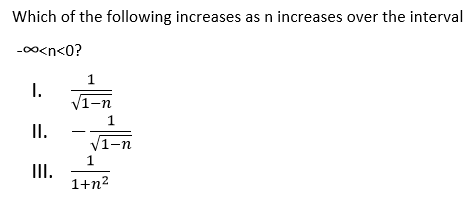I and II only

I and III only

II only

I only

II and III only

I and III only

Explanation: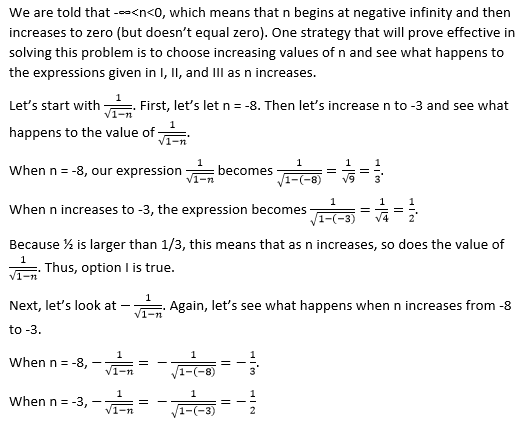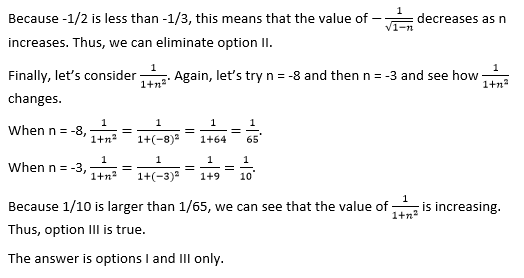### Example Question #7 : How To Order Fractions From Least To Greatest Or From Greatest To Least

Which of the following fractions is between 0.2 and 0.3?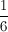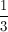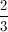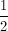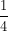Explanation: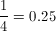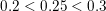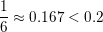The other three choices are larger than 0.3.

### Example Question #2 : How To Order Fractions From Least To Greatest Or From Greatest To Least

Which of the following fractions is the greatest?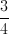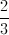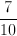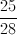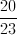Explanation:

The trick to this problem is to see that three of the choices have the numerator and denominator 3 units apart. The other two choices can be made three units apart as well- multiply bothandby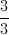to get them to be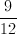and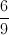, respectively.

The trick is that once all fractions are the same units apart in denominator and numerator, the biggest number is the largest fraction.

### Example Question #1 : How To Order Fractions From Least To Greatest Or From Greatest To Least

Order the following from least to greatest: three-fifths, seven-eighths, 0.5, sixteen-eighteenths, 97%, 17-fifths.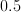, three-fifths, seven-eighths, sixteen-eighteenths,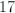-fifths,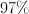three-fifths,,,  seven-eighths, sixteen-eighteenths,-fifths,, three-fifths, seven-eighths, sixteen-eighteenths,-fifths, three-fifths, sixteen-eighteenths, seven-eighths,-fifths, three-fifths, seven-eighths, sixteen-eighteenths,,-fifths, three-fifths, seven-eighths, sixteen-eighteenths,,-fifths

Explanation:

We need to order: three fifths, seven eights, 0.5, sixteen eighteenths, 97%, seventeen fifths.

Let us re-write each as a fraction: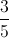,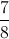,,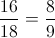,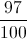, and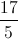. We can now easily order these: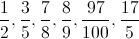### Example Question #10 : How To Order Fractions From Least To Greatest Or From Greatest To Least

Which of the following fractions is less than?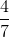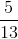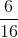Explanation:

The purpose of this question is to find understandable values of fractions, which must be done using a common scale. Since looking at the denominators in the answers shows that there are many different numbers, decimal values would be easier to use.

The decimal equivalencies are as follows: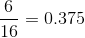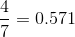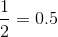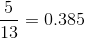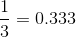Therefore, we know that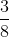is equal to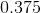and find that the fraction that is less than that is, which is equal to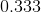.

← Previous 1

### All SAT Math Resources